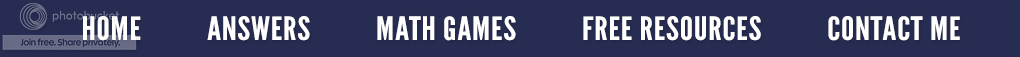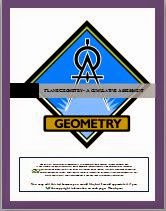### Are Trapezoids "Trapping" Your Students?

In a previous posting (Aliens and Trapezoids, July 7, 2011) I shared with you how I taught my students to remember the word trapezoid.  Today, I would like to talk about the characteristics of a trapezoid.As I search on Pinterest, I find quadrilaterals that look like the one on the right classified as "trapezoids".  Indeed they are, but this is a special kind of trapezoid because it has one set of equal sides, one line of symmetry and one set of parallel sides.  It is called an isosceles trapezoid.  Isosceles means "having two equal sides" just as an isosceles triangle has.
BUT to be a trapezoid, the only characteristic needed is one set of parallel sides.  Look at the red quadrilateral on the left.  It is a trapezoid because it has one set of parallel sides.  YET, students rarely see this kind except on those math tests that COUNT!  Why?  Because those trapezoids (and test writers) are out to "get" your students.  So think about it.  Are the trapezoids in your classroom trying to "trap" your students or can your students recognize a trapezoid even if it doesn't have symmetry?

--------------------------------------------------------------------------

I love teaching geometry, and therefore I have several geometry products for sale in my Teachers Pay Teachers Store.

Geometry Parodies - The four page handout includes 20 unusual definitions of geometric terms such as “A place where people are sent for committing crimes.” Each definition is a play on words or a parody.

Plane Geometry Test - This 100 point assessment is overplain geometry concepts and focuses on using and applying geometry. Measuring and categorizing angles, identifying lines, angles, quadrilaterals, etc., solving for circumference, using formulas, recognizing symmetry, and comparing using and applying geometry. Measuring and categorizing angles, identifying lines, angles, quadrilaterals, etc., solving for circumference, using formulas, recognizing symmetry, and comparing two quadrilaterals are included.

Plane Geometry Vocabulary Crossword - This puzzle is designed so that the student will practice and use geometric vocabulary. It is a free form crossword puzzle that features 25 different geometry terms. The 25 clues are in the form of definitions which emphasize points, lines, and angles.

Solid Geometry Test - This 100 point test is a summative assessment given at the end of the solids unit in our math book. It highlights using and applying formulas to find area, perimeter, circumference, surface area, and volume.

Solid Geometry Vocabulary Crossword - This crossword puzzle is designed to practice geometric vocabulary and recognize formulas. It is a free form crossword puzzle that features 23 different geometric terms or formulas. The 23 clues are in the form of definitions or a formula format which give emphasis to polyhedrons, circles, and formulas for area, surface area, and volume.